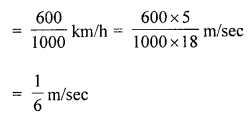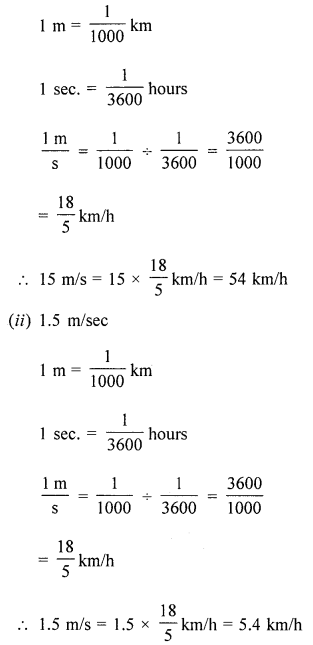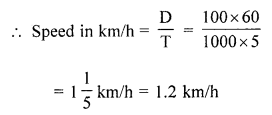## ML Aggarwal Class 7 Solutions for ICSE Maths Chapter 6 Ratio and Proportion Ex 6.4

Question 1.
Convert the following speeds into m/sec:
(i) 72 km/h
(ii) 9 km/h
(iii) 1.2 km/minutes
(iv) 600 m/hour
Solution:
(i) 72 km/h
1 km = 1000 m
and 1 h = 3600 sec.Question 2.
Convert the following speeds into km/h:
(i) 15 m/sec
(ii) 1.5 m/sec
Solution:
(i) 15 m/secQuestion 3.
Which is greater- the speed of 30 m/sec or 30 km/h?
Solution:
Which is greater
m/s = $$\frac { 18 }{ 5 }$$ km/h
30 km/h = $$\frac { 18 }{ 5 }$$ × 30 = 108 km/h
That means 108 km cover in 1 hour 30 m/sec is greater

Question 4.
An aeroplane is flying at a speed of 720 km/h.
(i) If the aerial distance between two cities is 1800 km, how much time will the aeroplane take in crossing these cities?
(ii) How much distance does the aeroplane cover in 40 minutes?
(iii) How far will it fly in 15 seconds?
Solution:
Speed of an aeroplane = 720 km/h
(i) Distance between two cities = 1800 km
Time taken = $$\frac { D }{ S }$$
= $$\frac { 1800 }{ 720 }$$ hours
= $$\frac { 5 }{ 2 }$$ hours
= 2$$\frac { 1 }{ 2 }$$ hours
(ii) Time = 40 min
= $$\frac { 40 }{ 60 }$$
= $$\frac { 2 }{ 3 }$$ h
Distance travelled = Time × Speed
= $$\frac { 2 }{ 3 }$$ × 720
= 480 km
(iii) Distance travelled in 15 sec = $$\frac { 15 }{ 3600 }$$ = $$\frac { 1 }{ 240 }$$ h
= $$\frac { 1 }{ 240 }$$ × 720
= 3 km

Question 5.
A dog is walking at a speed of 6 km/h.
(i) How much distance does it cover in 5 minutes?
(ii) How much time would it take to cover 200 metres?
Solution:
Speed of a dog = 6 km/h
(i) Distance travelled in 5 min. = $$\frac { 5 }{ 60 }$$ × 6 km
= $$\frac { 1 }{ 2 }$$ km
= 500 m
(ii) Time taken to cover 200 m
= $$\frac { 200 }{ 1000 }$$ km × $$\frac { 1 }{ 6 }$$ h
= $$\frac { 1 }{ 30 }$$ h
= $$\frac { 1 }{ 30 }$$ × 60
= 2 minutes

Question 6.
A swimming pool is 50 metres long. A boy can swim across the length and then return to his starting position in 5 minutes. What is his swimming speed in km/h?
Solution:
Length of a swimming pool = 50 m
A boy crosses it and then come back
Total distance covered = 50 × 2 = 100 m
Time taken = 5 minutesQuestion 7.
A bus takes 48 minutes to cover a certain distance when travelling at a speed of 50 km/h. How much time will it take to cover the same distance when travelling at a speed of 30 km/h?
Solution:
Speed of a bus = 50 km/h
Time taken = 48 minutes
Distance = Speed × Time
= 50 × $$\frac { 48 }{ 60 }$$ km = 40 km
In second time speed = 30 km/h
Time taken = $$\frac { D }{ S }$$
= $$\frac { 40 }{ 30 }$$
= $$\frac { 4 }{ 3 }$$ h
= 1 hours $$\frac { 1 }{ 3 }$$ min.
= 1 hours $$\frac { 1 }{ 3 }$$ × 60 mm.
= 1 hour 20 minutes